Fluid Mechanics homework Help at TutorEye

# Best Homework Help For Fluid Mechanics

## Fluid Mechanics:

Fluid Mechanics is a branch of physics, which deals with the motion of fluid and its consequences due to the external force and pressure. It  is the study of fluids in static and dynamic conditions. The motion of fluid is governed by the equation of classical mechanics.It’s application in a wide range of fields including mechanical, biomedical and astrophysics.

## Fluid Mechanics Sample Questions:

Question 1: In case of viscosity of fluid, __________ force is predominant in liquid.

c) Intermolecular           d) Ionic

Explanation: Due to strong cohesive force between liquid

Question 2: The specific weight of 30dm of diesel of specific gravity 0.6 is (dm stands for decimeter)

a) 11772          b) 11720

c) 5552             d) 5886

Explanation: Volume of diesel V =

Question 3: For a Non-Newtonian fluid, A is called ___________ in the expression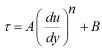a) Initial stress               b) Viscosity

c) Flow behaviour          d) Consistency index

Explanation: A - Consistency index

Question 4: During positive capillary rise (???? < 90°), adhesive force is ______ than cohesive force.

a) greater               b) equal

c) smaller               d) none of the above

Explanation: Adhesive force is greater than the cohesive force, which results in capillary rise.

Question 5: Which of the following is the correct expression for gauge pressure according to the hydrostatic law ?

a)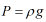b)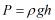c)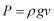d)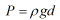Answer:  b)Explanation: Let the pressure acting vertically downward = P

Question 6: A glass tube is immersed in water having surface tension 0.0735 N/m. The radius of the tube when rise of water is restricted to 11 mm.

a) 2.55            b) 1.74

c) 1.33            d) 1.65

Explanation:  Given,

Question 7: For the flow to be irrotational

a)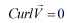b)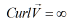c)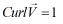d)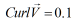Answer: a)Explanation: If curl V

Question 8: Mercury is used in barometer because of _________

a) High density                 b) Low vapour pressure

c) Both (a) and (b)             c) None

Answer: c) Both (a) and (b)

Explanation: d) Mercury is used,